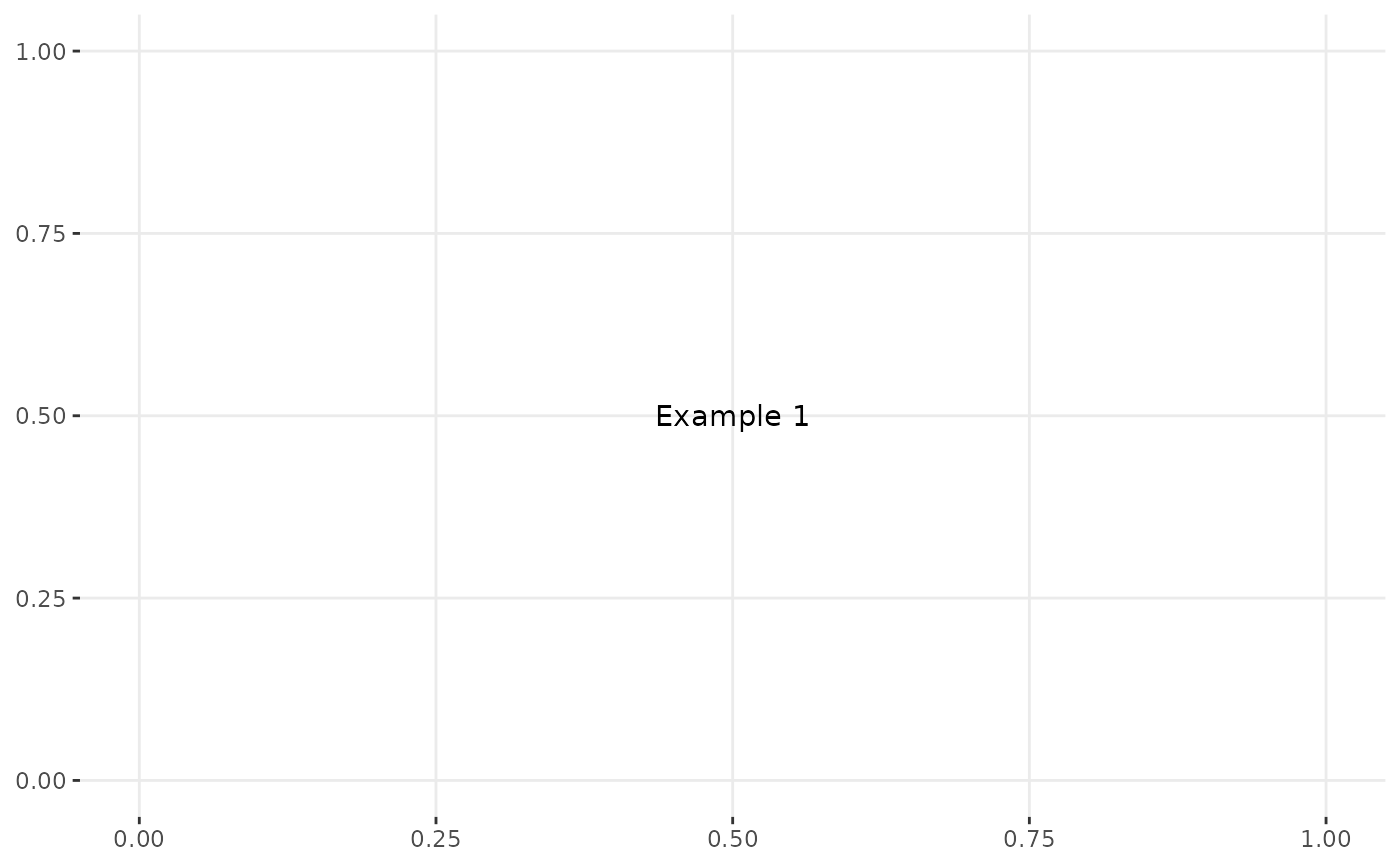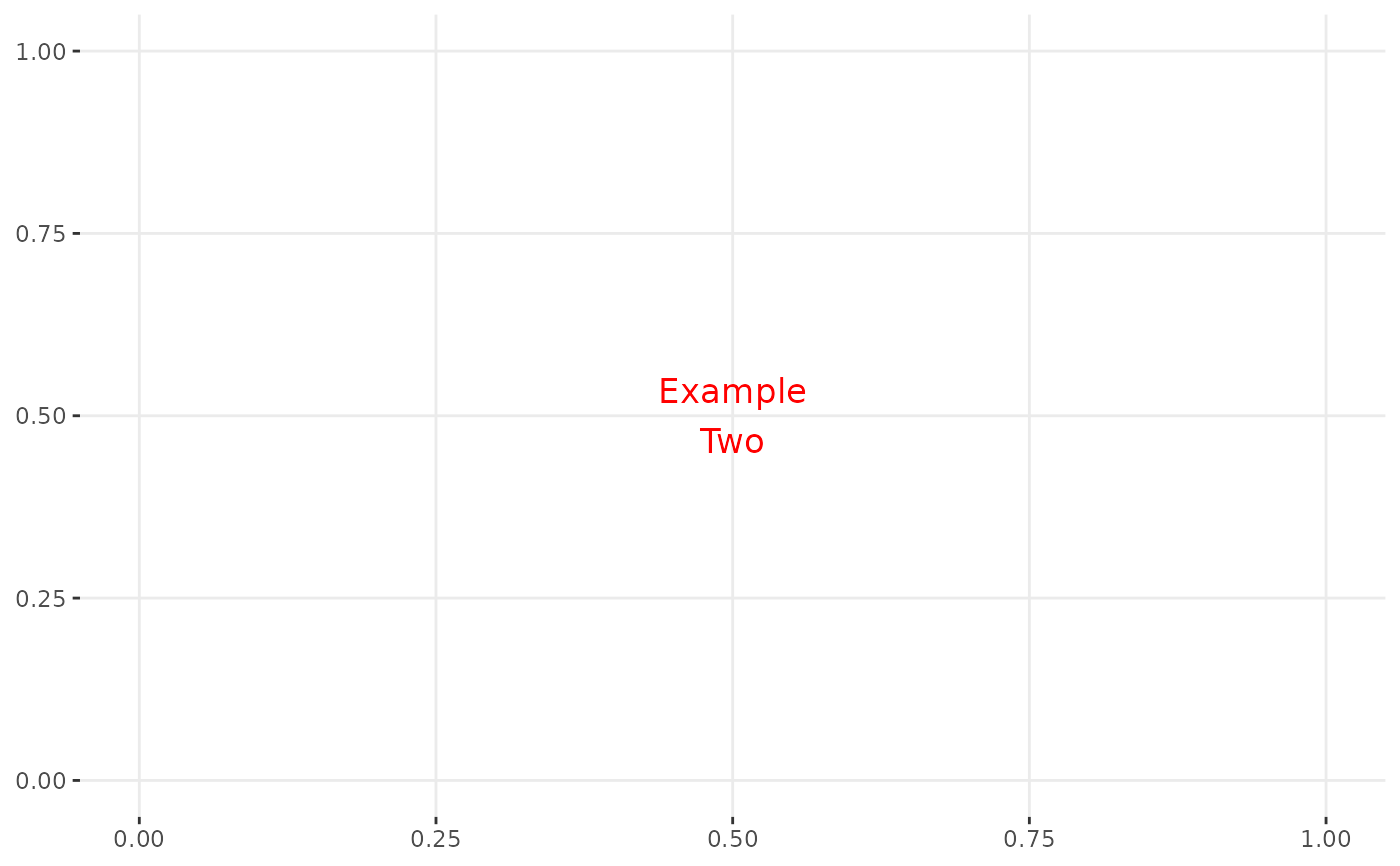Plot text for a plot.

ggally_text(
label,
mapping = ggplot2::aes(color = "black"),
xP = 0.5,
yP = 0.5,
xrange = c(0, 1),
yrange = c(0, 1),
...
)

## Arguments

label text that you want to appear aesthetics that don't relate to position (such as color) horizontal position percentage vertical position percentage range of the data around it. Only nice to have if plotting in a matrix range of the data around it. Only nice to have if plotting in a matrix other arguments for geom_text

Barret Schloerke

## Examples

# Small function to display plots only if it's interactive
p_ <- GGally::print_if_interactive

p_(ggally_text("Example 1"))p_(ggally_text("Example\nTwo", mapping = ggplot2::aes(size = 15), color = I("red")))